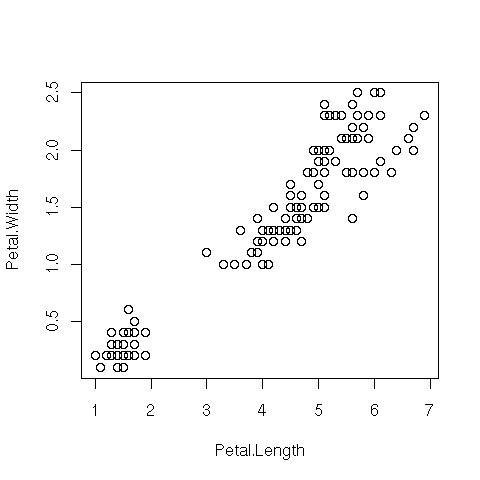DATA MINING
Desktop Survival Guide
by Graham Williams# Basic Plot

R's plot function provides the basic interface to the sophisticated graphics system. Given any object plot will endeavour to determine how to display the object. Other components of the graphic can then be built up using a variety of functions. As new components are added, they lay on top of what is already on the graphic, possibly occluding other components.

Standard types of plots include scatterplots, boxplots, barplots, histograms, and piecharts. Each can be quite simply generated through high level calls to R functions.

The simplest of plots is the scatterplot which displays the location of points on a two dimensional plot. Here we attach the common iris dataset and choose two variables to plot: Petal.Length and Petal.Width. The attach function allows the column names to be used without the normal `iris\$` prefix (by adding the dataset to the search path). A detach removes the object from the search pathThe resulting scatterplot illustrates some degree of correlation between these two variables, in that, generally speaking, for larger petal lengths, the petal width is also larger. We can also see two clear groups or clusters in this data: a cluster of entities with a petal length less than 2 and width less than about 0.6, and another group with petal length greater than about 3 and petal width greater than about 0.9.```attach(iris) plot(Petal.Length, Petal.Width) detach() ```

http://rattle.togaware.com/code/rplot-iris-scatter.R

If the dataset is only being used by the plot function and nowhere else then we could use with:

 ```with(iris, plot(Petal.Length, Petal.Width)) ```

Of course, we could simply use the full path to each column of data to be plotted:

 ```plot(iris\$Petal.Length, iris\$Petal.Width)) ```